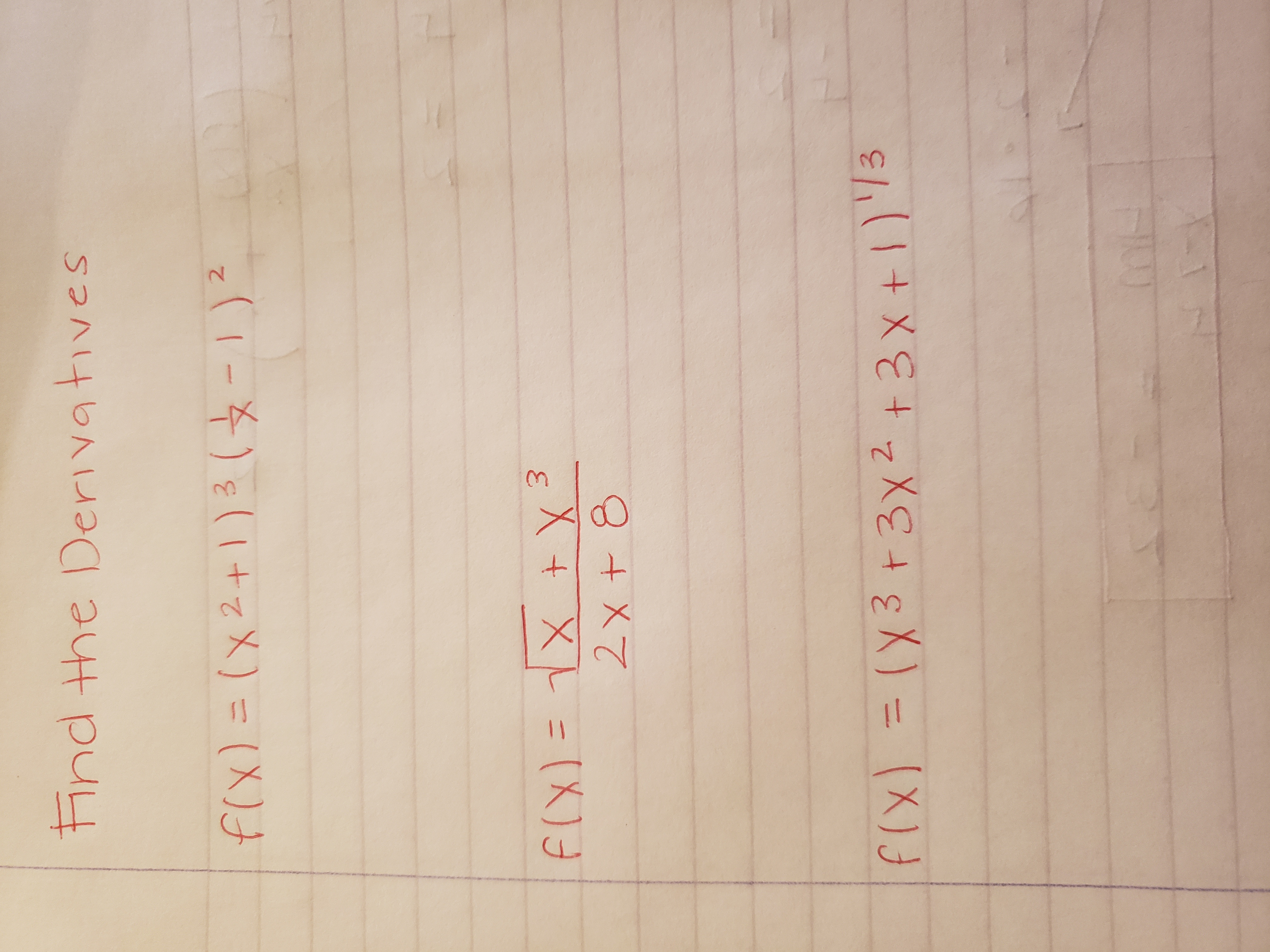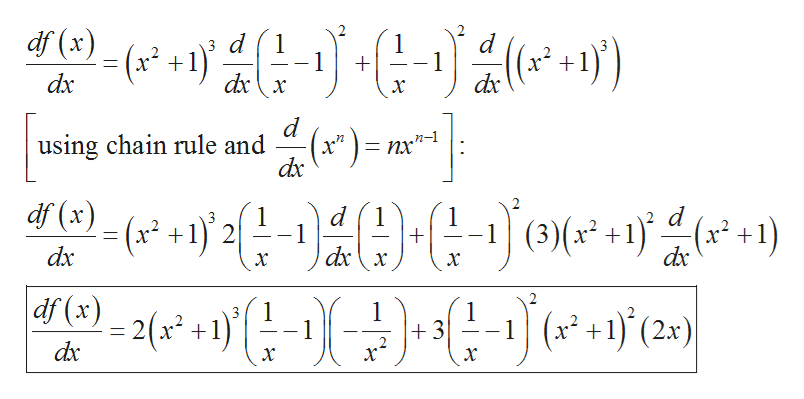# Hnd the DerivativesfCX)= (X2+1 )3(12F(X)=1X X32x+ 8f(x) = (X3 +3x2)3+3 xt

Question
1 viewshelp_outlineImage TranscriptioncloseHnd the Derivatives fCX)= (X2+1 )3(12 F(X)=1X X3 2x+ 8 f(x) = (X3 +3x2)3 +3 xt fullscreen
check_circle

Step 1

It is needed to find the derivatives of the given function.

The first function whose derivative is to find is:

Step 2

Use product rule to find the derivative:help_outlineImage Transcriptionclosedf (x) d d x dx 1 (a +1) dxx dx х d using chain rule and dx (x*) nrn- df (x) d1 d 1 -1 (3)(x +1)( 1) (x 1)'2 1 dxx _ dx dx х х ) df(x 3 1 1 +3 х 1 (x2+1) (2x) = 2( x^ +1 dx х х fullscreen
Step 3

The second function whose der...

### Want to see the full answer?

See Solution

#### Want to see this answer and more?

Solutions are written by subject experts who are available 24/7. Questions are typically answered within 1 hour.*

See Solution
*Response times may vary by subject and question.
Tagged in

### Derivative